# 为什么要神经网络

## 面对高维特征时的线性回归

``````y = m0 + m1 * x1 + m2 *x2 ... m_n * x_n
``````

``````y = m_0 + m_1 * x_1 + m_2 * x_2 + m_1_2 * x_1 * x_2 + m_1_1 * x_1^2 + m_2_2 * x_2^2 + .... + m_n_n * x_n^2
``````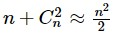## 图片数据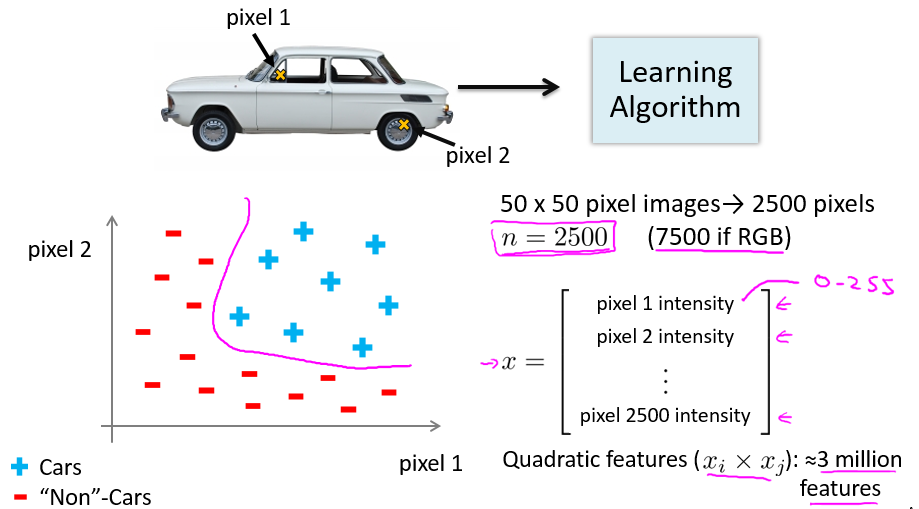# 神经网络定义

## 人体神经元模型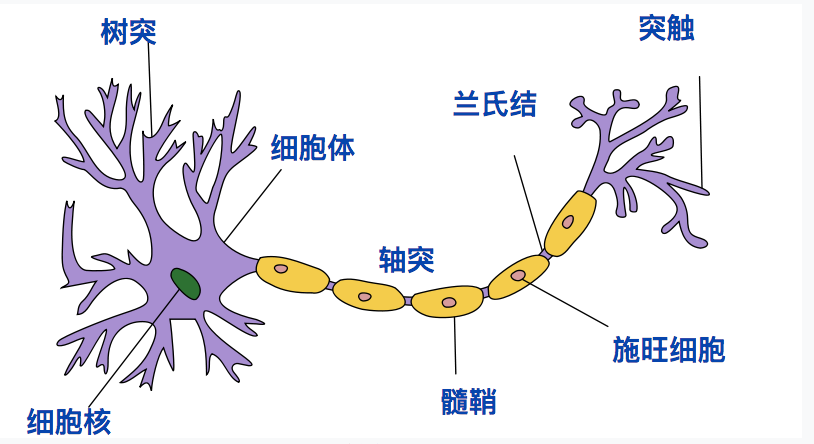• 接收区（receptive zone）：树突接收到输入信息。
• 触发区（trigger zone）：位于轴突和细胞体交接的地方，决定是否产生神经冲动。
• 传导区（conducting zone）：由轴突进行神经冲动的传递。
• 输出区（output zone）：神经冲动的目的就是要让神经末梢，突触的神经递质或电力释出，才能影响下一个接受的细胞（神经元、肌肉细胞或是腺体细胞），此称为突触传递。

## 人工神经网络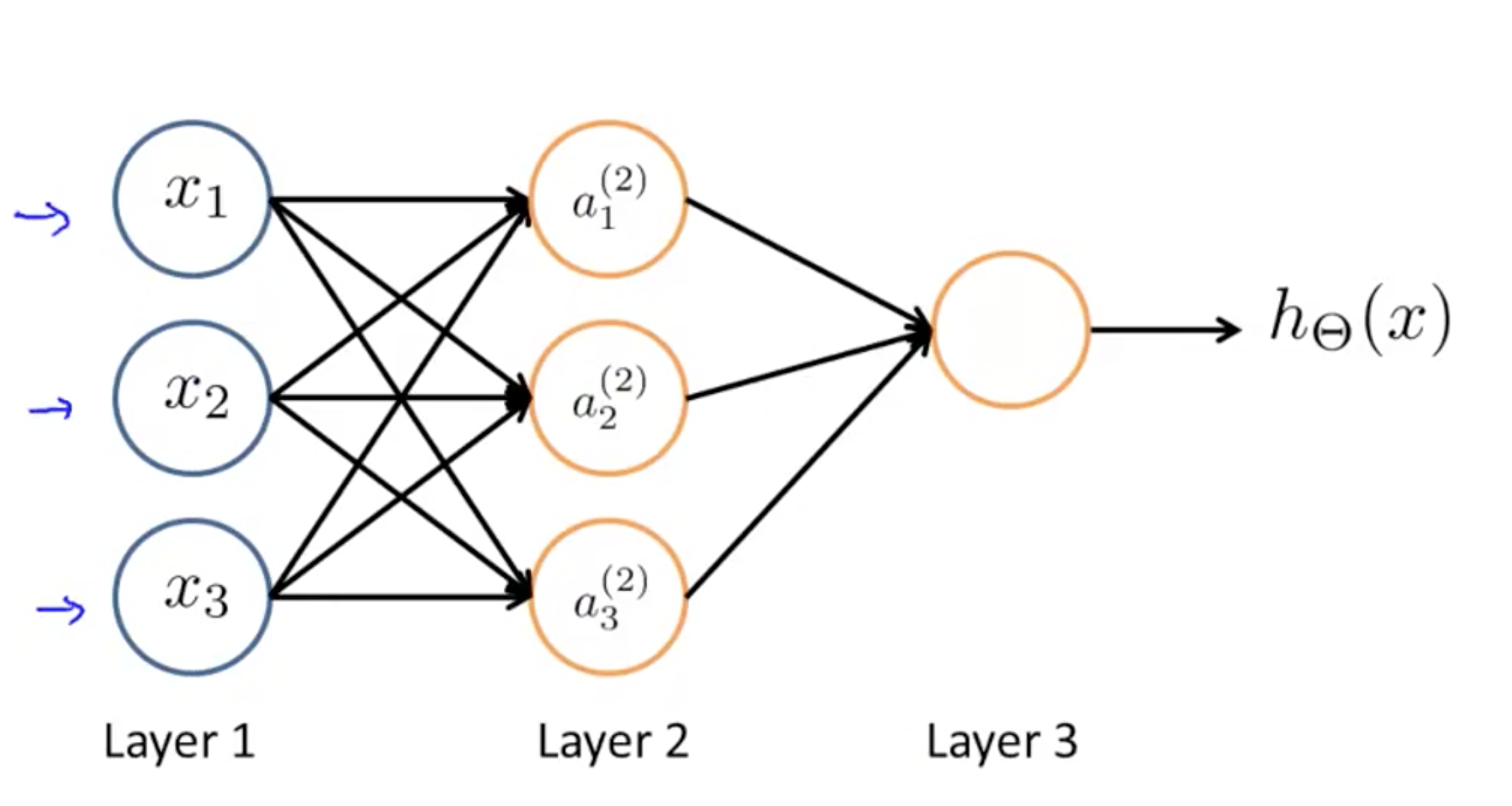• 输入层：输入层接收特征向量 x 。
• 输出层：输出层产出最终的预测 h 。
• 隐含层：隐含层介于输入层与输出层之间，之所以称之为隐含层，是因为当中产生的值并不像输入层使用的样本矩阵 X 或者输出层用到的标签矩阵 y 那样直接可见。

# 前向传播与反向传播

## 提前准备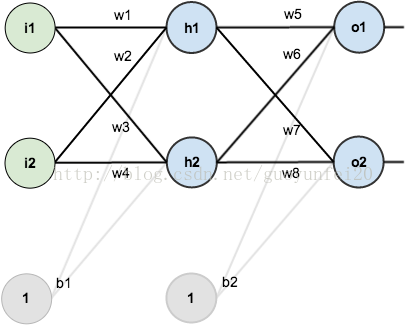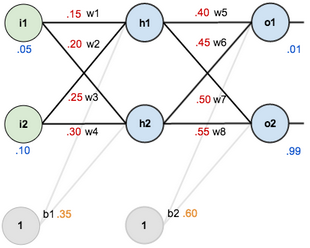## 前向传播

``````net_h1 = w_1 * i_1 + w_2 * i_2 + b1 * 1
``````

``````net_h1 = 0.15 * 0.05 + 0.2 * 0.1 + 0.35 * 1 = 0.3775
````````````net_h2 = 0.596884378
``````

``````net_O1 = w_5 * out_h1 + w_6 * out_h2 + b2 * 1
``````

``````net_O1 = 1.105905967
``````

``````out_O1 = 0.75136507
``````

``````out_O2 = 0.772928465
``````

## 统计误差``````E_O1 = 0.298371109
``````

``````E_O2 = 0.023560026
``````

``````E_total = E_O1 + E_O2 = 0.2983711109
``````

## 反向传播Content